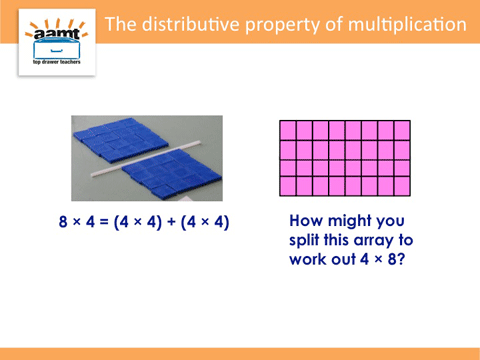Home > Mental computation > Big ideas > Generalising patterns and relationships > Distributive property of multiplication

# Distributive property of multiplication

The distributive property for multiplication splits one or both of the factors into two or more parts which can be multiplied separately and the partial products then added.

For example, to work out 6 $$\times$$ 8, split the 8 into 5 and 3 and then multiply each by 6. The equation would be (6 $$\times$$ 5) + (6 $$\times$$ 3), giving a product of 48 which is the same as for the original 6 $$\times$$ 8.For example, 8 $$\times$$ 16 can be thought of as (8 $$\times$$ 10) + (8 $$\times$$ 6).